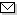Language:   Search:   ContactSearch and Browse the MSC 2000

Search:
Please note that in the second half of 2009, MSC2010 is deployed in the production of ZMATH and will become official standard in January 2010.

Enter a query and click »Search«...

32-XX Several complex variables and analytic spaces {For infinite-dimensional holomorphy, see 46G20, 58B12} → ZMATH
32Qxx Complex manifolds → ZMATH
32Q05 Negative curvature manifolds → ZMATH
32Q10 Positive curvature manifolds → ZMATH
32Q15 Kähler manifolds → ZMATH
32Q20 Kähler-Einstein manifolds [See also 53Cxx] → ZMATH
32Q25 Calabi-Yau theory → ZMATH
32Q28 Stein manifolds → ZMATH
32Q30 Uniformization → ZMATH
32Q35 Complex manifolds as subdomains of Euclidean space → ZMATH
32Q40 Embedding theorems → ZMATH
32Q45 Hyperbolic and Kobayashi hyperbolic manifolds → ZMATH
32Q55 Topological aspects of complex manifolds → ZMATH
32Q57 Classification theorems → ZMATH
32Q60 Almost complex manifolds → ZMATH
32Q65 Pseudoholomorphic curves → ZMATH
32Q99 None of the above, but in this section → ZMATH

NewsAbel prize 2010I. M. Gelfand 1913-2009MSC2010

Copyright © 2019 Zentralblatt MATH | European Mathematical Society | FIZ Karlsruhe | Heidelberg Academy of Sciences